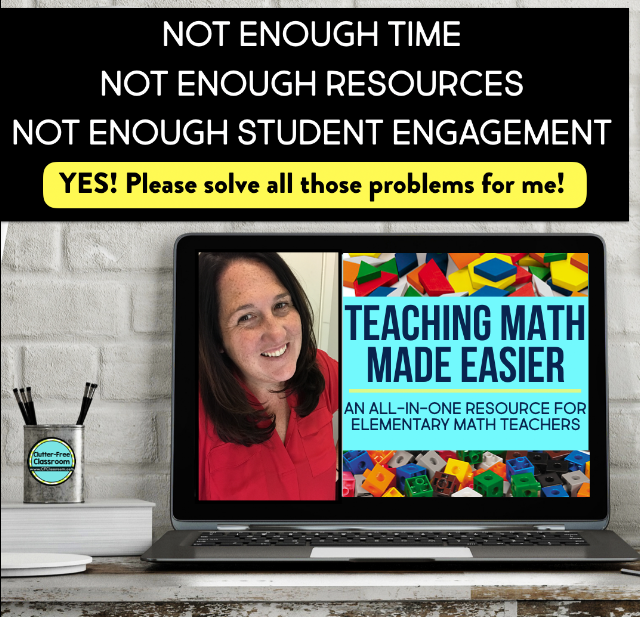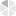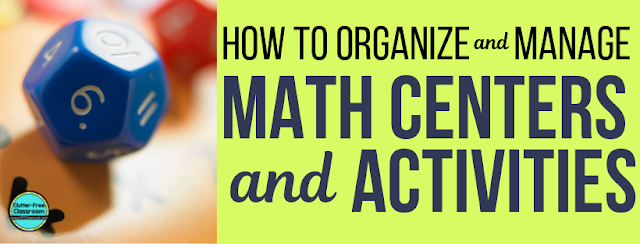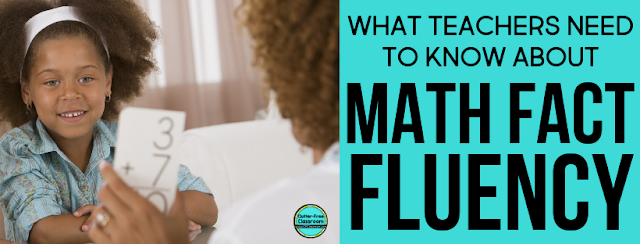# The Standards for Mathematical Practice

Have you heard acronyms like SMP, MPS, and NCTM used, and are unsure what they stand for or perhaps what they are? Read below to make sense of these acronyms and learn about what that the Standards for Mathematical Practice are, what the Mathematical Process Standards are for the TEKS, and how to make these standards kid-friendly.

What does standards for mathematical practice mean?
When you hear someone refer to the math practices or SMPs, they are referring to the Standards for Mathematical Practice, which are a set of eight standards that describe what a proficient mathematician can do.  These standards extend all the way from Kindergarten to twelfth grade.

What are the 8 Standards for Mathematical Practice for the CCSS?
1. Make sense of problems and persevere in solving them. Students need to be able to determine the meaning of a given problem, brainstorm possible strategies, and construct a plan of how they can solve. Throughout the problem solving process, they need to change their plan if needed and regularly ask themselves, “Does this make sense?”
2. Reason abstractly and quantitatively. Students need to be able to pull out important information from a given problem, create a representation, and consider the problem as a whole.
3. Construct viable arguments and critique the reasoning of others. Students need to be able to communicate their ideas and thinking clearly, while also being prepared to further explain and justify them with definitions and reasons.  In addition, they need to be able to analyze someone else’s argument, decide if it makes sense, and ask thoughtful questions for the purposes of clarifying and improving the person’s argument.
4. Model with mathematics. Students need to be able to represent problems using objects, drawings, diagrams, equations, tables, graphs, etc.  They then use these representations to help them solve a given problem.
5. Use appropriate tools strategically. Students need to be able to choose the best tools to help them solve problems. These tools include a pencil and paper, ruler, meter stick, measuring tape, calculator, hundreds chart, coins, base-ten blocks, three-dimensional shapes, tangrams, etc.
6. Attend to precision. Students need to be able to precisely communicate, measure, label, calculate, follow procedures, etc.
7. Look for and make use of structure. Students need to be able to identify patterns and make connections in order to understand properties and mathematical structures.
8. Look for and express regularity in repeated reasoning. Students need to be able to identify when calculations are repeated. In addition, they need to be able to identify similarities amongst problems, make and explain generalizations, and find shortcuts.

What are the Mathematical Process Standards (MPS) for TEKS?
The Mathematical Process Standards are part of the TEKS, which stands for Texas Essential Knowledge and Skills.  Unlike the eight standards for mathematical practice, these standards are not limited to math content.  They describe ways students should engage with math, science, and social studies content.  Students are expected to be able to do the following 7 skills:
1. Apply mathematics to problems arising in everyday life, society, and the workplace.
2. Use a problem-solving model that incorporates analyzing given information, formulating a plan or strategy, determining a solution, justifying the solution, and evaluating the problem-solving process and the reasonableness of the solution.
3. Select tools, including real objects, manipulatives, paper and pencil, and technology as appropriate, and techniques, including mental math, estimation, and number sense as appropriate, to solve problems.
4. Communicate mathematical ideas, reasoning, and their implications using multiple representations, including symbols, diagrams, graphs, and language as appropriate.
5. Create and use representations to organize, record, and communicate mathematical ideas.
6. Analyze mathematical relationships to connect and communicate mathematical ideas.
7. Display, explain, and justify mathematical ideas and arguments using precise mathematical language in written or oral communication.

As you can see, there are both similarities and differences amongst the Mathematical Process Standards and Standards for Mathematical Practice.

How do I make the standards kid-friendly?

It is essential to communicate the standards to students so they understand the goal or goals that they are working towards in a given lesson. Learning is most purposeful when standards are communicated both orally and in writing at the beginning of the lesson, referred back to during the lesson, and addressed again at the end of the lesson. When communicating these standards, it is critical that the teacher use kid-friendly language.  If the teacher does not use language that the students can easily understand, stating the standards becomes far less effective.  As a result, teachers should remember the 5 ideas below when making the standards kid-friendly.
1. Structure the standard as an “I can” statement.
2. Use simple, developmentally-appropriate language.
3. Utilize direct, active-voice language.
4. Limit the standard to one concise sentence.
5. Add a visual to support understanding when applicable.### READ ALL THE BLOG POSTS IN THE ELEMENTARY MATH SERIES:By the way, if you are not already subscribed to The Clutter-Free Classroom newsletter I encourage you sign up. Subscribers receive weekly tips for organizing and managing a classroom as well as exclusive free printables. You can sign up here.